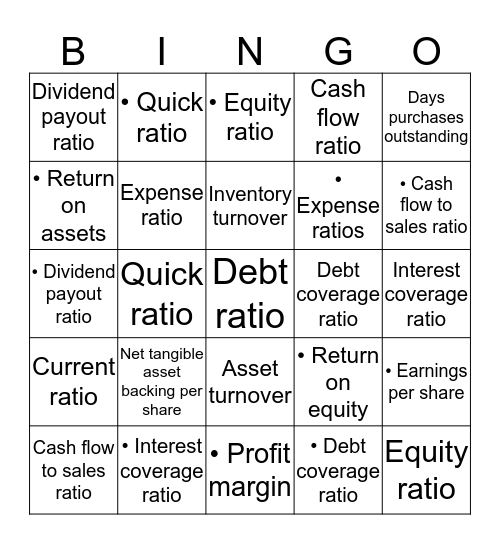Ratio AnalysisThis bingo card has a free space and 39 words: Return on equity, Return on assets, Profit margin, Expense ratio, Cash flow to sales ratio, Asset turnover, Inventory turnover, Days sales outstanding, Days purchases outstanding, Current ratio, Quick ratio, Cash flow ratio, Equity ratio, Interest coverage ratio, Debt coverage ratio, Net tangible asset backing per share, Earnings per share, Dividend payout ratio, Price earnings ratio, • Return on equity, • Return on assets, • Profit margin, • Expense ratios, • Cash flow to sales ratio, • Asset turnover, • Inventory turnover, • Days Sales Outstanding, • Days Purchases Outstanding, • Current ratio, • Quick ratio, • Cash flow ratio, • Debt ratio, • Equity ratio, • Interest coverage ratio, • Debt coverage ratio, • Net tangible asset backing per share, • Earnings per share, • Dividend payout ratio and • Price Earnings Ratio.

Play Online